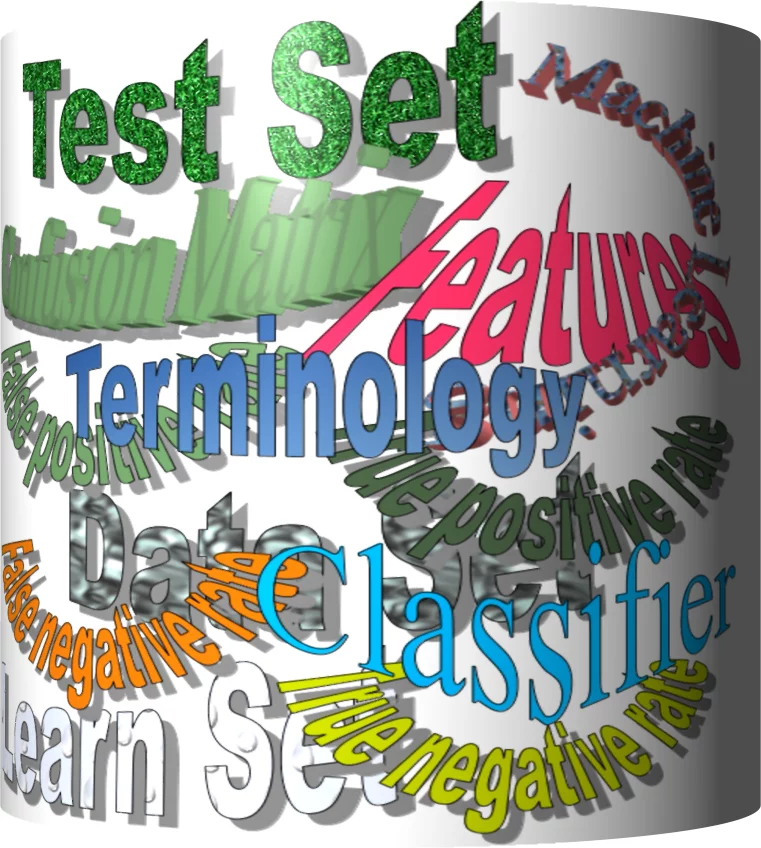## Machine Learning Terminology#### Classifier

A program or a function which maps from unlabeled instances to classes is called a classifier.

#### Confusion Matrix

A confusion matrix, also called a contingeny table or error matrix, is used to visualize the performance of a classifier.

The columns of the matrix represent the instances of the predicted classes and the rows represent the instances of the actual class. (Note: It can be the other way around as well.)

In the case of binary classification the table has 2 rows and 2 columns.

Example:

Confusion
Matrix
Predicted classes
male female
Actual
classes

male 42 8
female 18 32

This means that the classifier correctly predicted a male person in 42 cases and it wrongly predicted 8 male instances as female. It correctly predicted 32 instances as female. 18 cases had been wrongly predicted as male instead of female.

#### Accuracy (error rate)

Accuracy is a statistical measure which is defined as the quotient of correct predictions made by a classifier divided by the sum of predictions made by the classifier.

The classifier in our previous example predicted correctly predicted 42 male instances and 32 female instance.

Therefore, the accuracy can be calculated by:

accuracy = $(42 + 32) / (42 + 8 + 18 + 32)$

which is 0.72

Let's assume we have a classifier, which always predicts "female". We have an accuracy of 50 % in this case.

Confusion
Matrix
Predicted classes
male female
Actual
classes

male 0 50
female 0 50

We will demonstrate the so-called accuracy paradox.

A spam recogition classifier is described by the following confusion matrix:

Confusion
Matrix
Predicted classes
spam ham
Actual
classes

spam 4 1
ham 4 91

The accuracy of this classifier is (4 + 91) / 100, i.e. 95 %.

The following classifier predicts solely "ham" and has the same accuracy.

Confusion
Matrix
Predicted classes
spam ham
Actual
classes

spam 0 5
ham 0 95

The accuracy of this classifier is 95%, even though it is not capable of recognizing any spam at all.

#### Precision and Recall

Confusion
Matrix
Predicted classes
negative positive
Actual
classes

negative TN FP
positive FN TP

Accuracy: $(TN + TP)/(TN + TP + FN + FP)$

Precision: $TP / (TP + FP)$

Recall: $TP / (TP + FN)$

#### Supervised learning

The machine learning program is both given the input data and the corresponding labelling. This means that the learn data has to be labelled by a human being beforehand.

#### Unsupervised learning

No labels are provided to the learning algorithm. The algorithm has to figure out the a clustering of the input data.

#### Reinforcement learning

A computer program dynamically interacts with its environment. This means that the program receives positive and/or negative feedback to improve it performance.